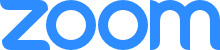# Omsk Algebraic Online Webinar

Upcoming meeting:

### "On the embedding of free nilpotent groups in partially commutative nilpotent groups."

Abstract. An algorithm is presented that allows one to calculate the maximum rank of a free nilpotent group of class k into a given partially commutative nilpotent group of class k.The meeting will be held using the Zoom platform. Join us via browser Or join us with Zoom Id: 812 2079 3393

Latest announcements:

### "Binary Lie algebras and its application to the theory of Binary Lie superalgebras."

Abstract. The tropical structures min-plus and max-plus are well-known and have many interesting applications.
By definition an algebra $$B$$ is binary-Lie algebra iff any two elements $$a,b\in B$$ generate a Lie subalgebra. A $${\bf Z}_2-$$graded algebra $$B=B_0\oplus B_1$$ is a binary-Lie superalgebra iff $$B\times \Gamma=B_0\otimes \Gamma_0\oplus B_1\otimes \Gamma_1$$ is a binary Lie algebra, where $$\Gamma=\Gamma_0\oplus \Gamma_1$$ is a Grassman algebra with natural gradation. We apply the theory of binary-Lie algebras for proving the following result.
Theorem. Let $$B=B_0\oplus B_1$$ be a simple binary-Lie superalgebra finite dimensional over the field $${\bf C}$$ of complex numbers. Then $$B_0$$ is a solvable algebra or $$B$$ is a Lie superalgebra.
This theorem reduced the problem (open yet!) of classification of a simple binary-Lie superalgebra finite dimensional over the field $${\bf C}$$ to the case where subalgebra $$B_0$$ is solvable.
This talk is based on the joint paper with A.Grishkov and I.Shestakov.

### "Tropical approach in cryptography."

Abstract. The tropical structures min-plus and max-plus are well-known and have many interesting applications.
In two papers “Tropical Cryptography” (2014) and “Tropical Cryptography II: extensions by homomorphisms” (2019), V. Shpilrain and D. Grigoriev suggested using tropical algebras as platforms for several cryptographic schemes in order to avoid linear algebra attacks. Another reason for their popularity is efficiency because multiplication is replaced by addition. Also, in “An application of different dioids in public key cryptography” (2014), M. Durcheva offered other cryptographic protocols based on tropical algebras and similar algebras. In recent years, other authors also developed some schemes in this area.
Many of these protocols were analyzed and attacks were developed by M. Kotov and A. Ushakov (2018), A. Muanalifah, and S. Sergeev (2020), D. Rudy and C. Monico (2020), S. Isaac and D. Kahrobaei (2021), K. Ahmed, S. Pal, and R. Mohan (2022).
Recently, we analyzed one of Durcheva’s protocols and implemented a successful attack (joint work with Ivan Buchinskiy and Alexander Treier).
In this talk, I will review the current status of tropical cryptography (protocols, methods to analyze them, and attacks) and discuss our new attack.

### "Generic complexity of the word problem in some semigroups."

Abstract. Generic algorithms decide problems for almost all inputs and ignore remaining rare inputs. I.Kapovich, A.Myasnikov, P.Schupp and V.Shpilrain in 2003 suggested a generic algorithm, which decides the word problem in a wide class of finitely generated groups, including classical groups with undecidable word problem. D. Won in 2008 proposed a generic algorithm for finitely defined semigroups with so called balanced presentation. In particular, classical Markov-Post, Tseitin and Makanin semigroups with undecidable word problems, all have balanced representation. In 2019 M. Volkov at the Sverdlovsk semigroup seminar asked me about generic decidability of the word problem in every semigroup with one relation. For the classical decidability this is a well known and still open Adjan problem. In this talk I will present a generic algorithm for the word problem in a wide class of finitely generated semigroups, including balanced semigroups and semigroups with one relation.

### "Embedding of Malcev and alternative algebras."

Abstract. We continue studying associative f-representations, which was begun in A. I. Kornev, I.P. Shestakov, On associative representations of non-associative algebras, J. Algebra Appl.,17, No. 3, 1850051 (2018). We are interested in finding identities that an algebra satisfies if it has a faithful f-representation. We introduce the notions of g-associative and g-Lie algebras and we prove that any alternative (Malcev) algebra can be embedded into some g-associative (g-Lie) algebra. We show that any Malcev algebra can be embedded as a commutator subalgebra in some g-associative for two different polynomials g.